The Pythagoras FigureThe Pythagorean Diagram problem
Divide the above shape into identical triangles.  How many triangles are needed???

My Pythagorean problem was very popular.  33600 Triangles -- Mark Thompson, Michael Reid, Patrick Hamlyn.  Bill Daly -- 5376 Triangles.  1344 Triangles -- Joe Logic, Al Zimmermann, Livio Zucca.  Only Joseph DeVincentis sent me the construction below, with 336 triangles (1/2, 2/3, 5/6).  Can anyone prove this is minimal, or find a construction with fewer congruent triangles?  Definitely not a trivial problem.Joseph has made a nice puzzle based the classic 3-4-5 figure.  Using 9 3-4-5 triangles and 2 unit squares, make the triangle+squares figure.

material added august 24th

Can the whole thing be divided into 56 identical triangles?  Yes, it can!  Wei-Hwa Huang found an incredible solution.  Michael Reid found it next, calling it 'really surprising'.  Now that you know it is possible, can you figure out how it is done?  If you prove it impossible, recheck your assumptions.  It's okay to ignore all internal lines.

Roger Phillips found a 4-piece dissection of the Pythagorean Figure into a 7x8 rectangle.  On the topic of dissections, a French site by Alain Rousseau has beautiful animations of famous dissections.

material added august 28th

Livio Zucca solved the 56 triangle problem, and wrote an article about it.  Here are the solutions sent to me by Wei-Hwa Huang and Michael Reid.  Joseph DeVincentis also solved it.  Wei-Hwa solved it by trying to prove that 336 was a minimal solution.  To be thorough, he bisected the angles of a 3-4-5 triangle.  When he noticed a relation to the 1-2 triangle (Pi/2 + ArcTan[3/4] = ArcTan), he found the 56 triangle solution.  (I personally find it incredible that a 5000 year old figure has yielded such a wonderful new puzzle)The Pythagorean Figure in 56 triangles by Huang and Reid.
The 1-2 triangles have a lot of nice properties, so perhaps they can make nice polyform sets.  It turns out that there are 13 ways to put two of these triangles together, based on all the ways they seem to fit together.  What to call them?  I suggested HemiDominoes, and Deminoes (Demi meaning half).  Andrew Clarke suggested polydoms (dom = half a domino), and I like that better.  Here are the 13 didoms.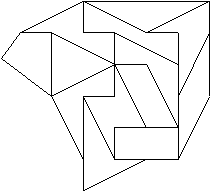The 13 didoms -- the ways to put two 1-2 triangles together.
Livio Zucca has much more material on these.  Here's one of his challenges -- make a dog shape with the 13 pieces.  Lovely -- I'm gonna have to solve this one.Livio Zucca's didom doggie
A problem -- can two sets of didoms + four triangles cover the Pythagorean Diagram?  If so, I think it would make a lovely set.  Do let me know if you find a solution for this.  Can twelve of the pieces make an equilateral octagon?  Or half a dodecagon?  Can two sets make a dodecagon?

For those that want to try generalizing the problem (how many triangles for the 5-12-13 figure?), two identities may be useful (n>m)
Tan[ 1/2 ArcTan[(2 m n)/(n2 - m2)] ] = m/n
Tan[ 1/2 ArcTan[(n2 - m2)/(2 m n)] ] = (n-m)/(n+m)
For integers m and n, {n2-m2, 2mn, n2+m2}is a pythagorean triangle.  For m=1, n=2, you'll get {3, 4, 5}. I'll add a diagram so that this isn't completely mystifing.

Michael Reid has found a dissection with a different triangle.

Brendan Owen and Roel Huisman both found that two sets of didoms + triangles can cover the Pythagorean figure.  I've been playing with the pieces, and think they are a lot of fun.  So, I'm making 16 of them as packages.I'm going for a work of art with these.  The pieces will be a quarter inch thick.  The whole package will be a solid 5/8 inches thick, and will include a fitted lid.  I'll be peeling all the pieces, which is why I'm making only 16 of them.  Pythagoras will be laser-etched as you see.  Cut squares and a 3-4-5 triangle will be under the pieces, with gridlines etched on the squares.  I'm working on a booklet to include with the pieces.  The two sets (one set = didoms+2 triangles) will be in two very clear but distinguishable colors.  Let me know if you want one of these packages.  Wei-Hwa Huang and Roel Huisman have already earned them.

Brendan Owen solved one of my idle questions:  Can two sets of didoms make a dodecagon?  The answer is YES!  For the extraordinary solution, again visit Livio Zucca's site.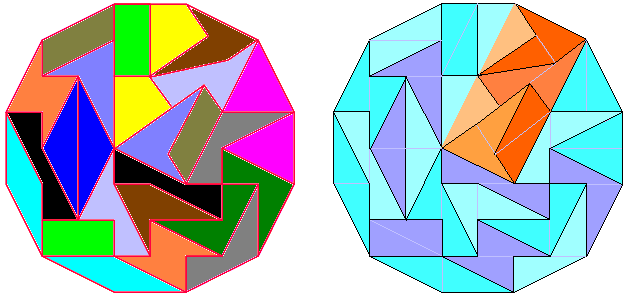Another development with the 1-2 triangles are the Dom-Sliced pentominoes.  Roel Huisman investigated them after looking at my site.  I was thinking of looking at them myself (really!), but he did first, and they turned out to be a superior polyform set.  You can take a look at them at Livio Zucca's site and at Andrew Clarke's site.  The complete set of 36 pieces will make a perfect square of size 12x12.  Theoretically, they can also make a 13x13 square with 5 squares inside, each with a side of sqrt(5).  Can a tilted X pentomino be centered in a 13x13 square with the dom sliced pentominoes? Mail me if you solve this.

Roel Huismen shows that a 7x22 rectangle can be made with the DSP set and two squares.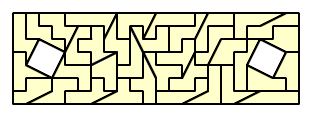Rectangle by Roel Huisman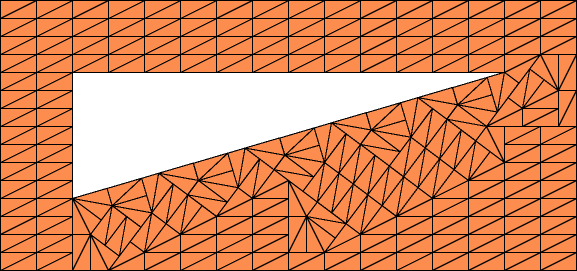Wei-Hwa Huang found that the 7-24-25 triangle can be outlined with 1-2 triangles (see above).

The mathematical recreations community has voted that the interesting new polyform will be call polydoms.  Polyformist Andrew Clarke coined the term.  A few weeks of work has revealed a lot about these pieces.  Two sets of didoms can make a dodecagon.  Add 4 doms, and you can make the 3-4-5 triangle figure.  Roel Huisman surprised me by using the same set to make a rectangle.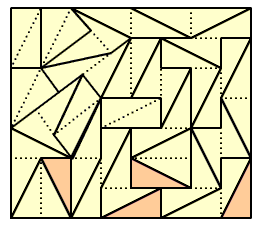Rectangle by Roel Huisman
But this set is yielding many surprises!  Livio Zucca has made a 4x13 rectangle with two didom sets!  Perhaps you can find it, now that you know it is possible.  Adding four doms, he made a hexagon with sides 4,5,5,4,5,5.  With help from Mario Ricciardi and Brendan Owen he has discovered much more.  Take a look at his site!  Admire the Big Pizza problem.  He gives an excellent problem there.  With 28 kites and 22 bricks, make a 10x10 square.  My own words on the Pythagoras Figure problem, Didoms, solvers, and commentary have been moved here, if you'd like to see even more.  Sixteen sets have been cut, but I'm still not sure of the price.  Three of the sets are still available, if you want one.  Write me.

Roger Phillips studied the general problem:
Haven't got as far as trying any tilings yet, but I can prove that for many (including the 5-12-13) it's not possible.

First, a definition: for the right triangle (n^2 - m^2), 2nm, (n^2+m^2) let's call the n,m right triangle the "root" triangle. Thus for every complex number z = n + im with integer coefficients n > m > 0, z represents the root triangle forming an angle A with the positive real axis, and z^2 = (n^2 - m^2) + i2nm represents the larger triangle, whose angle is 2A.

The area of the large triangle is nm(n^2 - m^2) = 2(n^2 - m^2)(area of root triangle), so the root area always divides the large triangle area. To tile the Pythagorean figure with the root triangle, we need its area to divide the three squares, which have a combined area of 2(n^2 + m^2)^2. So we need the following to be an integer:
2(n^2 + m^2)^2/(nm/2)
= 4(n^4 + 2n^2m^2 + m^4)/(nm)
= 8nm + 4(n^4 + m^4)/(nm)
So we need nm to divide
4(n^4 + m^4)
= 4(n - m)^4 + 4(n^3m - 6n^2m^2 + 4nm^3)
Since nm divides the second term, we need nm to divide 4(n - m)^4.

For the 3-4-5 triangle, (n,m) = (2,1) and 2 does divide 4. But this is the only triangle whose root is of the form (m + 1, m) (ie 3-4-5, 5-12-13, 7-24-25, ...) which can be tiled by its root triangle, as for these we need m(m + 1) to divide 4, which only has an integer solution for m = 1.
For triangles with root triangles of the form (m + 2, m) (ie 8-6-10, 12-16-20, 16-30-34, ...) we need m(m + 2) to divide 64, which (noting that all factors of 64 are powers of 2) only has a solution when m = 2.
For n - m = 3, we need m(m + 3) to divide 324, which has solutions for m = 1 and 3.
--
Roger Phillips